## Tamilnadu Samacheer Kalvi 11th Maths Solutions Chapter 2 Basic Algebra Ex 2.10

Determine the region in the plane determined by the inequalities:

Question 1.
x ≤ 3y, x ≥ y
Solution:
Given in equation are x ≤ 3y,x ≥ y
Suppose x = 3y ⇒ $$\frac{x}{3}=$$ = y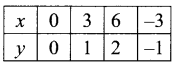If x = y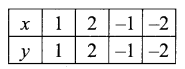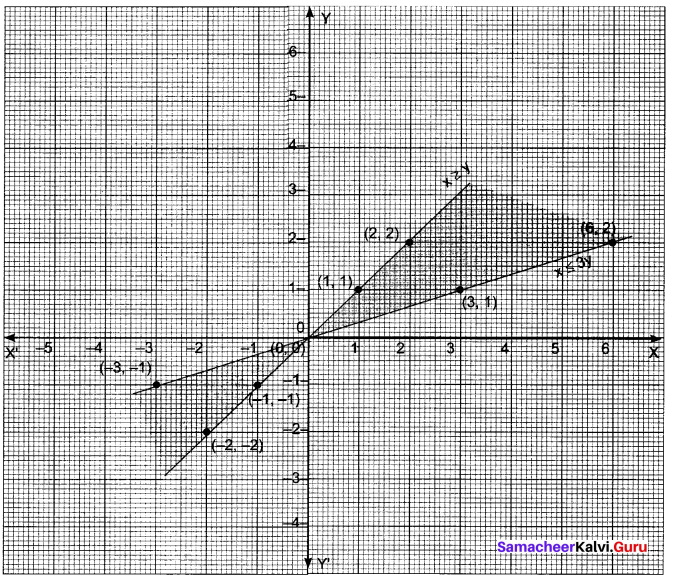Question 2.
y ≥ 2x, -2x + 3y ≤ 6
Solution:
Suppose y = 2x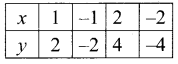-2x + 3y = 6
-2x = 6 – 3y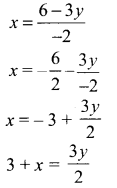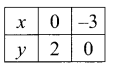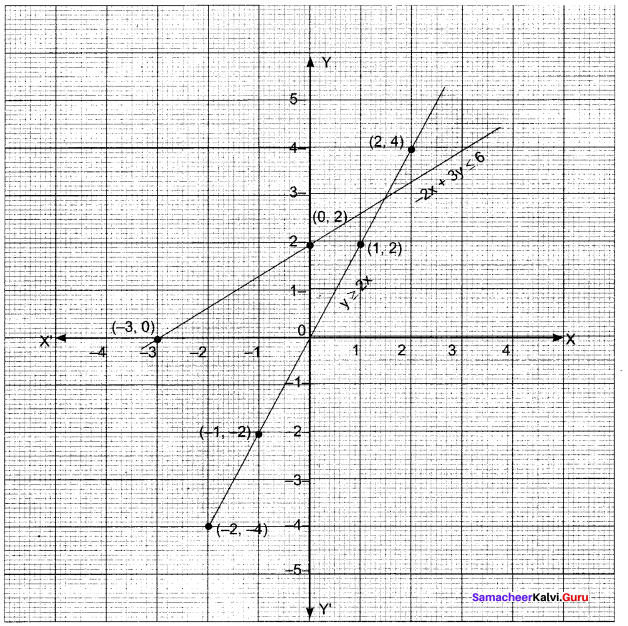Question 3.
3x + 5y ≥ 45, x ≥ 0, y ≥ 0.
Solution:
If 3x + 5y = 45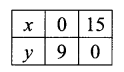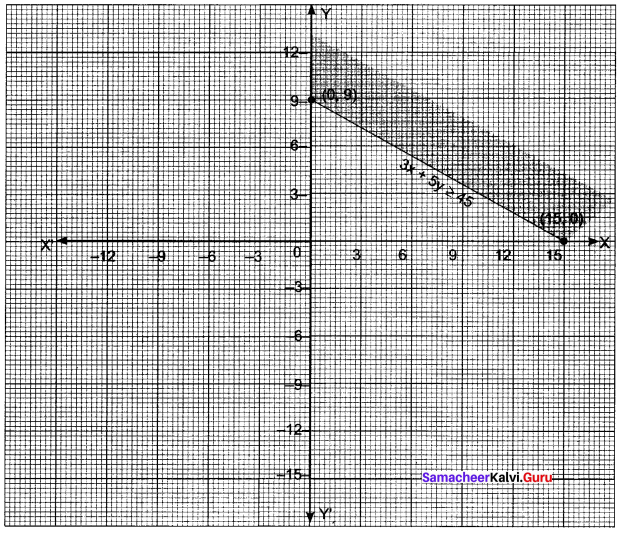x ≥ 0 is nothing but the positive portion of Y-axis and y ≥ 0 is the positive portion of X-axis.
Shaded region is the required portions.

Question 4.
2x + 3y ≤ 35, y ≥ 2, x ≥ 5
Solution:
If 2x + 3y = 35 then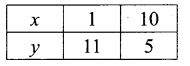y = 2 is a line parallel to X-axis at a distance 2 units
x = 5 is a line parallel to Y-axis at a distance of 5 units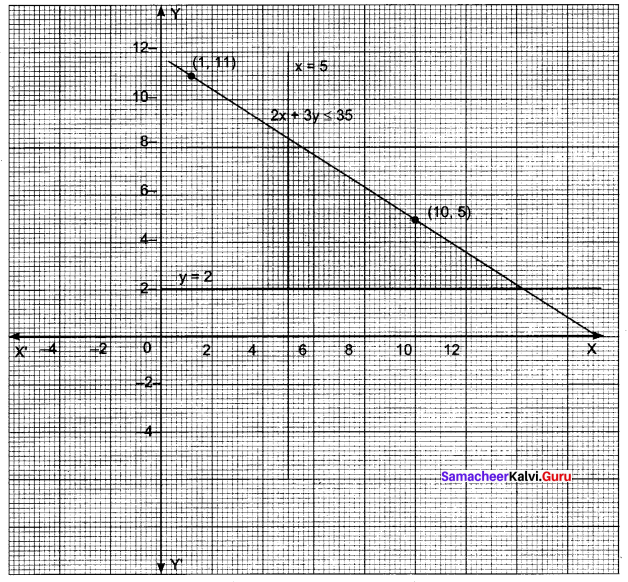The required region is below 2x + 3y = 35, above y = 2 and to the right of x = 5Question 5.
2x + 3y ≤ 6, x + 4y ≤ 4, x ≥ 0, y ≥ 0.
Solution:
If 2x + 3y = 6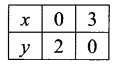x + 4y = 4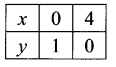x ≥ 0, y ≥ 0 represents the area in the 1 quadrant.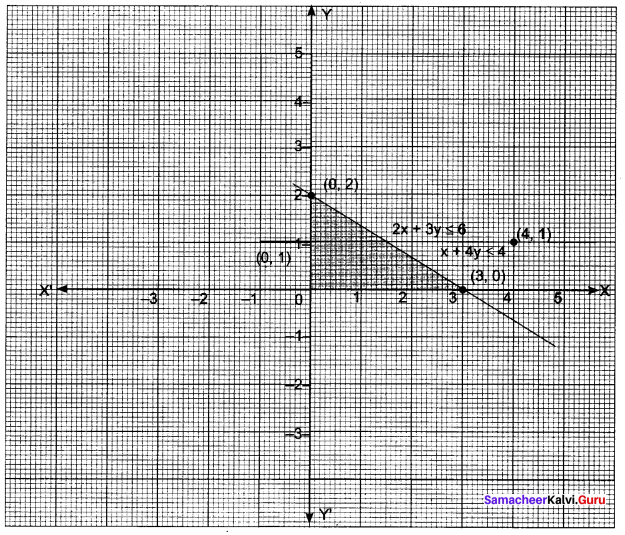The required region is below 2x + 3y = 6 and below x + 4y = 4 bounded by x-axis and y-axis.

Question 6.
x – 2y ≥ 0, 2x – y ≤ -2, x ≥ 0, y ≥ 0
Solution:
If x – 2y = 0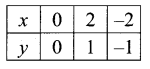2x – y = -2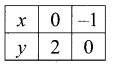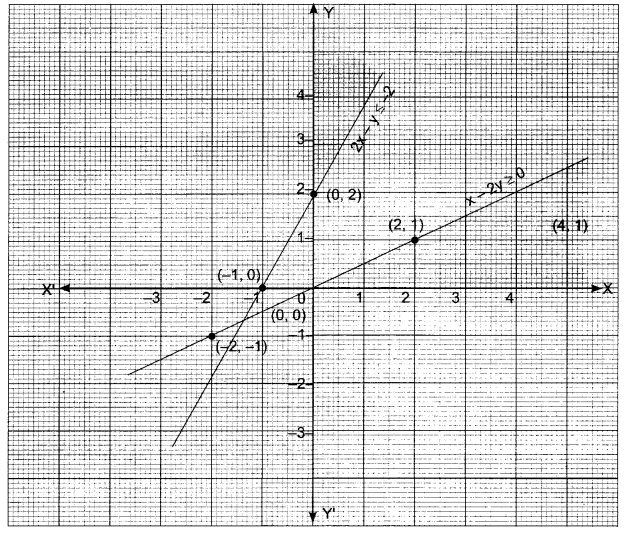x ≥ 0, y ≥ 0 represents the portion in the 1 quadrant only.

Question 7.
2x + y ≥ 8, x + 2y ≥ 8, x + y ≤ 6.
Solution:
2x + y = 8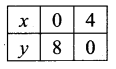x + 2y = 8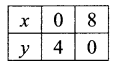x + y = 6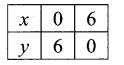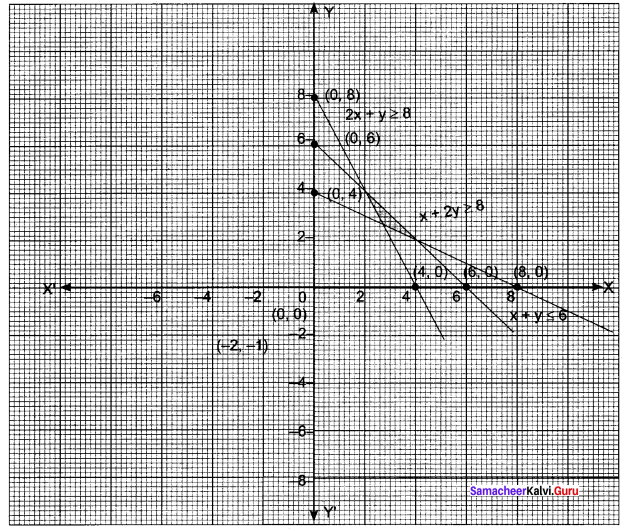### Samacheer Kalvi 11th Maths Solutions Chapter 2 Basic Algebra Ex 2.10 Additional Questions

Question 1.
3x + 2y ≤ 12, x ≥ 1, y ≥ 2
Solution:
The given inequality is 3x + 2y ≤ 12.
Draw the graph of the line 3x + 2y ≤ 12
Table of values satisfying the equation
3x + 2y ≤ 12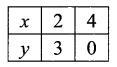Putting (0, 0) in the given inequation, we have 3 × 0 + 2 × 0 ≤ 12
∴ Half plane of 3x + 2y ≤ 12 is towards origin
Also the given inequality is x ≥ 1.
Draw the graph of the line x = 1.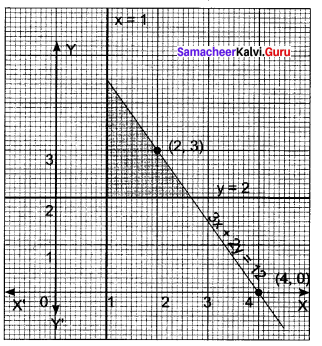Putting (0, 0) in the given inequation, we have 0 ≥ 1 which is false.
∴ Half plane of x ≥ 1 is away from origin.
The given inequality is y ≥ 2.
Putting (0, 0) in the given inequation, we have 0 ≥ 2 which is false.
∴ Half plane of y ≥ 2 is away from origin.Question 2.
x + y ≥ 4, 2x – y > 0
Solution:
The given inequality is x + y ≥ 4.
Draw the graph of the line x + y = 4.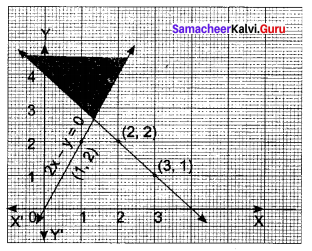Table of values satisfying the equation
x + y = 4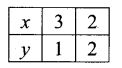Putting (0, 0) in the given inequation, we have 0 + 0 ≥ 4 ⇒ 0 ≥ 4, which is false.
∴ Half plane of x + y ≥ 4 is away from origin.
Also the given inequality is 2x – y > 0.
Draw the graph of the line 2x – y = 0.
Table of values satisfying the equation
2x – y = 0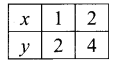Putting (3, 0) in the given inequation, we have 2 × 3 – 0 > 0 ⇒ 6 > 0, which is true.
∴ Half plane of 2x – y > 0 containing (3, 0)

Question 3.
x + y ≤ 9, y > x, x ≥ 0
Solution:
The given inequality is x + y ≤ 9.
Draw the graph of the line x + y = 9.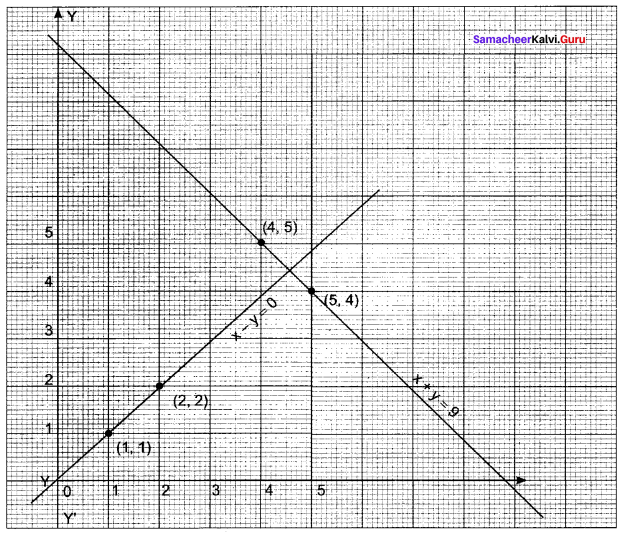Table of values satisfying the equation
x + y = 9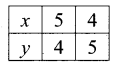Putting (0, 0) in the given inequation, we have 0 + 0 ≤ 9⇒ 0 ≤ 9, is towards is origin.
∴ Half plane of x + y ≤ 9 is away from origin.
Also the given inequality is x – y < 0.
Draw the graph of the line x -y = 0.
Table of values satisfying the equation
x – y = 0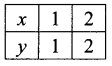Putting (0, 3) in the given inequation, we have 0 – 3 – 0 < 0 ⇒ -3 < 0, which is true.
∴ Half plane of x – y < 0 containing the points (0, 3).Question 4.
5x + 4y ≤ 20, x ≥ 1, y ≥ 2
Solution:
The given inequality is 5x + 4y ≤ 20.
Draw the graph of the line 5x + 4y = 20.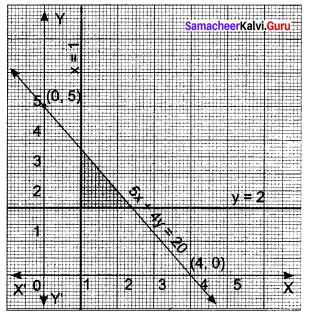Table of values satisfying the equation
5x + 4y = 20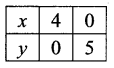Putting (0, 0) in the given inequation, we have 5 × 0 + 4 × 0 ≤ 20 ⇒ 0 ≤ 20, which is true.
∴ Half plane of 5x + 4y ≤ 20 is away from origin.
Also the given inequality is x ≥ 1.
Draw the graph of the line x = 1.
Putting (0, 0) in the given inequation, we have 0 ≥ 1, which is false.
∴ Half plane of x ≥ 1 is y ≥ 2.
Draw the graph of the line y = 2.
Putting (0, 0) in the given inequation, we have 0 ≥ 2, which is false.
∴ Half plane of y ≥ 2 is away from origin.Question 5.
3x +4y ≤ 60, x + 3y ≤ 30, x ≥ 0, y ≥ 0
Solution:
The given inequality is 3x + 4y ≤ 60.
Draw the graph of the line 3x + 4y = 60.
Table of values satisfying the equation
3x + 4y = 60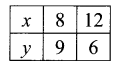Putting (0, 0) in the given inequation, we have 3 × 0 + 4 × 0 ≤ 60 ⇒ 0 ≤ 60, which is true.
∴ Half plane of 3x + 4y ≤ 60 is towards origin.
Also the given inequality is x + 3y ≤ 30.
Draw the graph of the line x + 3y = 30.
Table of values satisfying the equation .
x + 3y = 30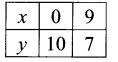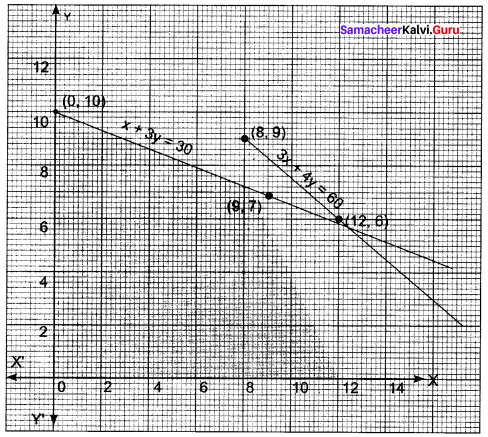Putting (0, 0) in the given inequation, we have 0 + 3 × 0 ≤ 30 ⇒ 0 ≤ 30, which is true.
∴ Half plane of x + 3y ≤ 30 is towards origin.